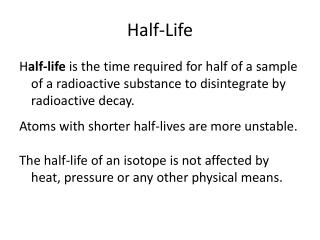DownloadDownload PresentationHalf-Life

Half-Life

Télécharger la présentationHalf-Life

- - - - - - - - - - - - - - - - - - - - - - - - - - - E N D - - - - - - - - - - - - - - - - - - - - - - - - - - -
Presentation Transcript

1. Half-Life Half-life is the time required for half of a sample of a radioactive substance to disintegrate by radioactive decay. Atoms with shorter half-lives are more unstable. The half-life of an isotope is not affected by heat, pressure or any other physical means.

2. Half-life is used by scientists to predict the age of an object. • The isotope C-14 is used to date materials that were once living or came from living things. • C-14 has a half life of 5715 yrs. This means that after 5715 yrs, a 100 gram sample of C-14 will be half C-14 and half some other isotope. After 11430 yrs, the sample will be 25 g C-14 and 75 grams of some other isotope.

3. Half-life Problems • In many problems, you will be given the total (elapsed) time and the length of one half-life. You have to determine the number of half-lives before working the problem. • To find the number of half-lives divide the elapsed time by the length of one half-live. • Ex. If one half-life is 8 days, and 24 days have gone by, how many half-lives have occurred?

4. Half-life Problems • Number of half-lives will be represented by n • Original mass of substance will be mi • Final mass of substance will be mf • Formula: mf = --------- mi 2n

5. 1. How much of a 100 g sample of Rn-222 is left after 15.2 days if its half life is 3.8 days? First find the number of half-lives that have occurred. Divide 15.2 days by 3.8 days to get the number of half lives: Givens: Hint: n =number of half lives, mi= original mass mf = final mass

6. 1. How much of a 100 g sample of Rn-222 is left after 15.2 days if its half life is 3.8 days? First find the number of half-lives that have occurred. Divide 15.2 days by 3.8 days to get the number of half lives: 4 Givens: n = 4 mi = 100 g mf = ? mi Formula mf = -------- mf = -------- 2n

7. 1. How much of a 100 g sample of Rn-222 is left after 15.2 days if its half life is 3.8 days? First find the number of half-lives that have occurred. Divide 15.2 days by 3.8 days to get the number of half lives: 4 Givens: n = 4 mi = 100 g mf = ? mi Formula mf = -------- mf = -------- = -------- = 2n 100 g 16 100 g 24

8. 2. How much of a 200 g sample of I-131 is left after 16 days if its half life is 8 days? First find the number of half-lives that have occurred Givens: n = mi = mf = ? Formula : mf = -------- n =number of half lives, mi = original mass mf = final mass

9. How much of a 200 g sample of I-131 is left after 16 days if its half life is 8 days? First find the number of half-lives that have occurred Givens: n = 2 mi = 200 mf = ? Formula : mf = -------- mf = ----------- = n =number of half lives, mi = original mass mf = final mass mi 2n

10. 2. How much of a 200 g sample of I-131 is left after 16 days if its half life is 8 days? First find the number of half-lives that have occurred Givens: n = 2 mi = 200g mf = ? Formula : mf = -------- mf = ----------- = n =number of half lives, mi = original mass mf = final mass mi 2n 200g 22 200g 4

11. To Find Original Amount • Rearrange the basic equation so that mi is by itself on one side of the equals sign. • mi = mf x 2n • 3. The half life of Iodine-131 is 8 days. If after 24 days there are 6.25 grams of Iodine-131 left. How much was in the original sample? n = mf = mi =

12. 4. If the half life of a radioactive sample is 4.0 days and 8 grams is left after 20 days, how much did you start with?

13. 6. How many half-lives will it take for 160 grams to be reduced to 40 grams?

14. Sometimes you will be given the percent or fractional amount that remains or has decayed instead of the number of days. • To find the number of half lives multiply by ½ until you get the percent or fractional amount that remains • EX: If the problems says that 1/32 remains, multiply ½ x ½ x ½ x ½ x ½ until you get 1/32. The number of half lives will be 5. • Ex: If the problem says that 7/8 has decayed, you must determine that 1/8 remains, then multiply ½ x ½ x ½ until you get 1/8. The number of half-lives will be 3.

15. Ra-226 has a half life of 1599 years. How long would it take 15/16 of a Ra-226 sample to decay? First determine how much remains: 16/16-15/16 = 1/16 Then determine number of half-lives by multiplying ½ by itself until you get 1/16. ½ x ½ x ½ x ½ = 1/16 Count the number of ½s, so 4 half-lives have gone by. Multiply 4 x the half life of 1599 years: 4 x 1599 = 6396 years